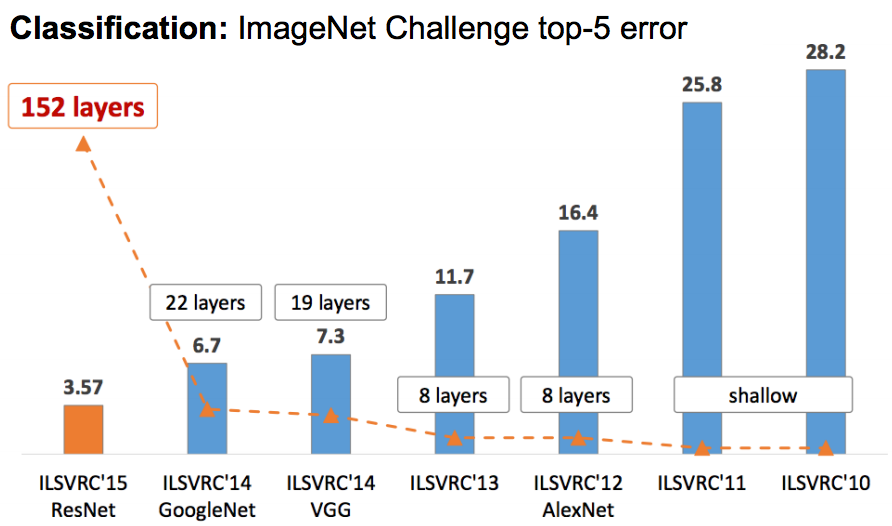# CNN Architectures — LeNet, AlexNet, VGG, GoogLeNet and ResNet

In my previous blog post, explained about my understanding of Convolution Neural Network (CNN). In this post, I am going to detailing about convolution parameters and various CNN architectures used in ImageNet challenge. ImageNet has been running an annual competition in visual recognition (ILSVRC# — “ ImageNet Large Scale Vision Recognition Challenge” from 2010 onwards) where participants are provided with 1.4 millions of images. Below are some popular CNN architectures won in ILSVRC competitions.

• LeNet-5
• AlexNet
• VGGNet
• ResNet

Before delve into see the above mentioned details, let us see why convolution and pooling layers are used in front of FC layers (Fully Connected Layer) and how convolution, pooling layers is being calculated in order to filtering essential features in an input image.

Why convolution layers are used before the fully connected layers?

Generally, convolution and pooling layers act as giant filters. Imagine an image 224 x 224 x 3 pixels and FC layer as a direct first hidden layer with 100,000 perceptrons, the total number of connections will be 224*224*3*100000=15,02,800,000 =>15 billion parameters which is impossible to process. Convolution and max pooling layers can actually help to reduce some features in the image which are may not required to train.

Convolution Parameters

In convolution layer, it accepts a volume of size W x H x D and requires four hyper parameters as follows:

• Number of filters → K
• Spatial Extent → Fw, Fh (Filter width, Filter height)
• Stride → Sw, Sh (Stride width, Stride height)
• Padding → P

To calculate receptive field, the formula is as follows,

To calculate pooling layer, the formula is as follows,

• OM → Output Matrix
• IM → Input Matrix
• P → Padding
• F → Filter
• S → Stride

By applying above receptive and pooling calculation formulas, the convolutions, pooling and feature maps outputs are derived. The key operations in a CNN,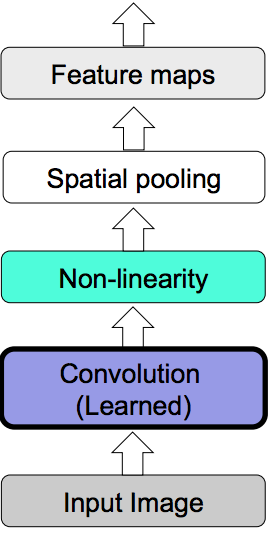Figure 2 : CNN Key Operation (Source : R.Fergus, Y.LeCun)

LeNet-5

In this classical neural network architecture successfully used on MNIST handwritten digit recogniser patterns. Below is the LeNet-5 architecture model.

LeNet-5 receives an input image of 32 x 32 x 1 (Greyscale image) and goal was to recognise handwritten digit patterns. It uses 5 x 5 filter and with stride is 1. By applying the above receptive field calculation formula and the output volume result is 28 x 28. The derivation is in below,

• W x H → 32 x 32 (Width x Height)
• F(w x h) → 5 x 5 (Filter)
• S → 1 (Stride)
• P → 0 (Pooling)

Next layer is a pooling layer, to calculate pooling layer in the above LeNet-5 architecture, the derivation as follows in below,

• IM → 28 (Input Matrix → Convolution output volume., Refer above derivation output)
• P → 0 (Pooling)
• S → 1 (Stride)

Finally, it goes to fully connected (FC Layer) layer with 120 nodes and followed by another FC Layer with 84 nodes. It uses Sigmoid or Tanh nonlinearity functions. The output variable Yhat with 10 possible values from digits 0 to 9. It is trained on MNIST digit dataset with 60K training examples.

AlexNet

It starts with 227 x 227 x 3 images and the next convolution layer applies 96 of 11 x 11 filter with stride of 4. The output volume reduce its dimension by 55 x 55. Next layer is a pooling layer which applies max pool by 3 x 3 filter along with stride 2. It goes on and finally reaches FC layer with 9216 parameter and the next two FC layers with 4096 node each. At the end, it uses Softmax function with 1000 output classes. It has 60 million parameters.

Some of the highlights in AlexNet Architecture:

• It uses ReLU activation function instead Sigmoid or Tanh functions. It speed by more than 5 times faster with same accuracy
• It uses “Dropout” instead of regularisation to deal with overfitting. But the training time is doubled with dropout ratio of 0.5
• More data and bigger model with 7 hidden layers, 650K units and 60M parameters.

VGG-16

VGG-16 is a simpler architecture model, since its not using much hyper parameters. It always uses 3 x 3 filters with stride of 1 in convolution layer and uses SAME padding in pooling layers 2 x 2 with stride of 2.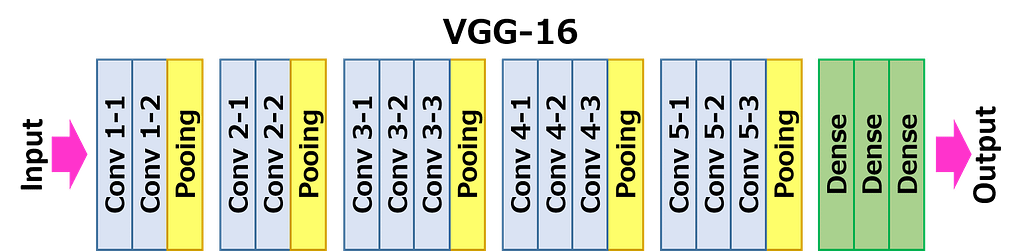Figure 5 : VGG-16 → Source

The winner of ILSVRC 2014 and the GoogLeNet architecture is also known as Inception Module. It goes deeper in parallel paths with different receptive field sizes and it achieved a top-5 error rate with of 6.67%.

This architecture consists of 22 layer in deep. It reduces the number of parameters from 60 million (AlexNet) to 4 million. The alternate view of this architecture in a tabular format in below

ResNet (2015)

The winner of ILSRVC 2015, it also called as Residual Neural Network (ResNet) by Kaiming. This architecture introduced a concept called “skip connections”. Typically, the input matrix calculates in two linear transformation with ReLU activation function. In Residual network, it directly copy the input matrix to the second transformation output and sum the output in final ReLU function.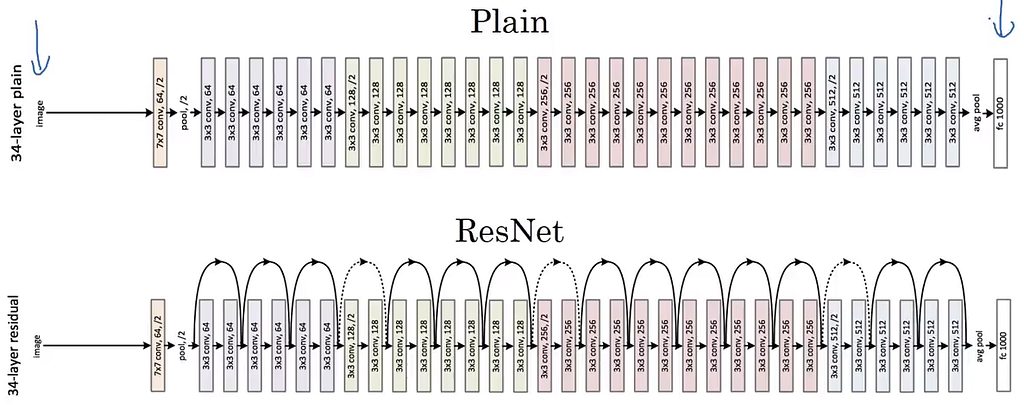Figure 7 : ResNet Architecture comparison with plain. Source

The overall summary table of various CNN architecture models in below.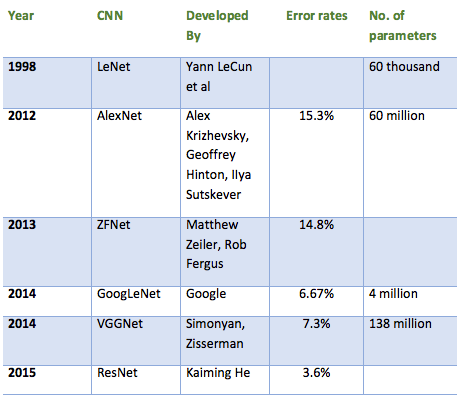Table 2 : ILSVRC competition CNN architecture models

References:Source: Deep Learning on Medium